# Tag: topology

Last time we discovered that the mental picture to view prime numbers as knots in $S^3$ was first dreamed up by David Mumford. Today, we’ll focus on where and when this happened.

3. When did Mazur write his unpublished preprint?

According to his own website, Barry Mazur did write the paper Remarks on the Alexander polynomial in 1963 or 1964. A quick look at the references gives us a coarse lower- and upper-estimate.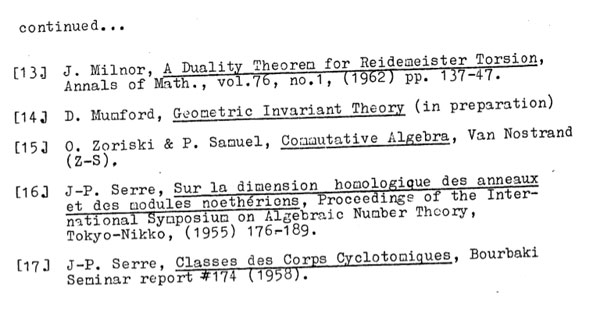Apart from a paper by Iwasawa and one by Milnor, all references predate 1962 giving us a lower-bound. More interesting is reference (14) to David Mumford’s Geometric Invariant Theory (GIT) which was first published in 1965 and is referred to as ‘in preparation’, so the paper was written no later than 1965. If we look a bit closer we see than some GIT-references are very preciseindicating that Mazur must have had the final version of GIT to consult, making it rather difficult to believe that the preprint was written late 1963 or early 1964.

Mazur’s dating of the preprint is probably based on this penciled note on the frontpage of the only surviving copy of the preprintIt reads : “Date from about 63/64, H.R. Morton”. Hugh Morton of Liverpool University confirms that it is indeed his writing on the preprint.

Further, he told me that early 64 Christopher Zeeman held a Topology Symposium in Cambridge UK, where Hugh was a graduate student at the time and, as far as he could recall, Mazur attended that conference and gave him the preprint on that occasion, whence the 63/64 dating. Hugh kindly offered to double-check this with Terry Wall who cannot remember Mazur attending that particular conference.

In fact, we will see that a more correct dating of the Mazur-preprint will be : late 1964 or early 1965.

4. The birthday : July 10th 1964

Clearly, Mumford’s insight predates the Mazur-preprint. In the first section, Mazur mentions ‘Grothendieck cohomology groups’ rather than ‘Etale cohomology groups’.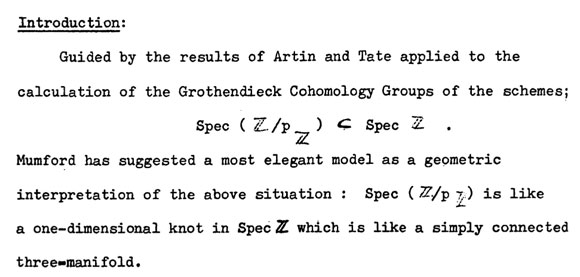At the time, Artin’s seminar notes on Grothendieck topologies (spring 1962) were widely distributed, and Artin and Grothendieck were in the process of developing etale cohomology in their Paris 1963/64 seminar SGA 4, while Mumford was working on GIT in Harvard.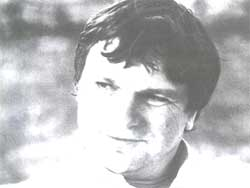Mike Artin, David Mumford and Jean-Louis Verdier all attended the Woods Hole conference from july 6 till july 31 1964, famous for producing the Atiyah-Bott fixed point theorem (according to Fulton first proved by Verdier at the conference).

Etale cohomology was a hot topic at that conference. On july 10th there were three talks, Artin spoke on ‘Etale cohomology of schemes’, Verdier on ‘A duality theorem in the etale cohomology of schemes’ and John Tate on ‘Etale cohomology over number fields’.

After a first week of talks, more informal seminars were organized, including the Atiyah-Bott seminar leading to the ‘Woods hole duality theorem’ and one by Lubin-Tate and Serre on elliptic curves and formal groups. Two seminars adressed Etale Cohomology.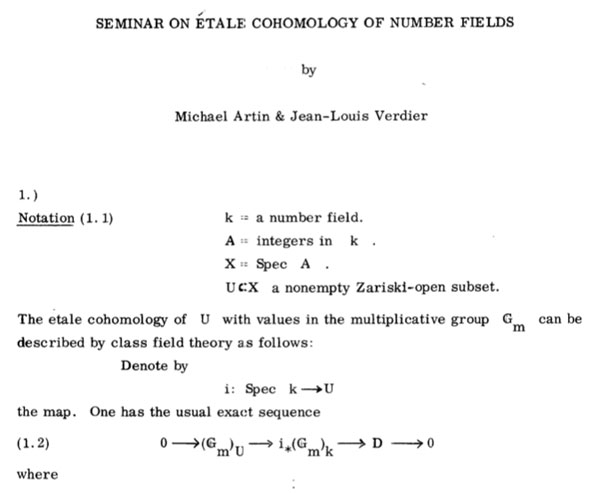Artin and Verdier ran a seminar on the etale cohomology of number fields leading to their duality result, and, three young turks : Daniel Quillen, Steve Kleiman and Robin Hartshorne ran a Baby Seminar on Etale cohomology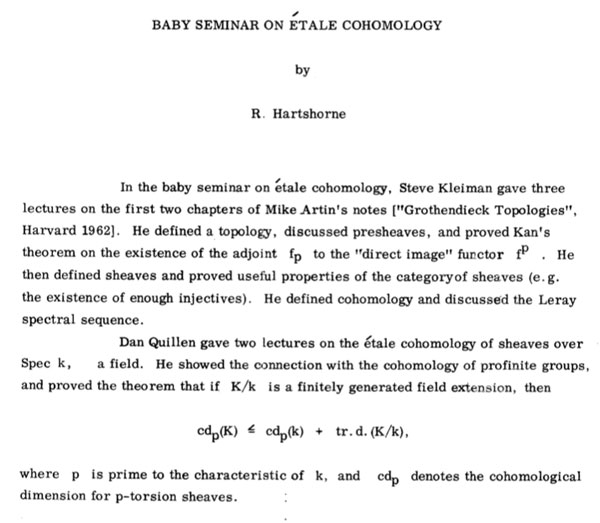Probably it is safe to say that the talks by Artin, Verdier and Tate on July 10th sparked the primes=knots idea, and if not then, a couple of days later.

5. The birthplace : the Whitney Estate

The ‘Woods Hole’ conference took place at the Whitney Estate and all the lectures took place in the rustic rooms of the main building and the participants (and their families) were housed in rented cottages in the neighborhood, for the duration of the summer.

The only picture i managed to find from the Whitney house comes from a rather surprising source : Gardeners and Caretakers ofWoods Hole. Anyway, here it is :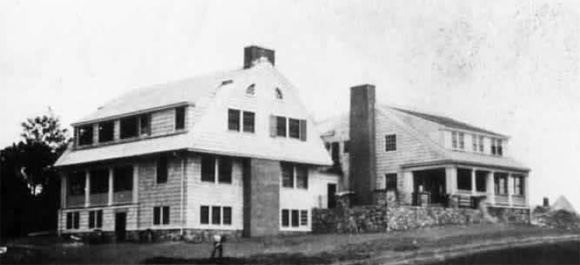Probably, the knots=primes analogy was first dreamed up inside, or in the immediate neighborhood, on a walk to or from the cottages, overlooking the harbor.

One of the more surprising analogies around is that prime numbers can be viewed as knots in the 3-sphere $S^3$. The motivation behind it is that the (etale) fundamental group of $\pmb{spec}(\mathbb{Z}/(p))$ is equal to (the completion) of the fundamental group of a circle $S^1$ and that the embedding

$\pmb{spec}(\mathbb{Z}/(p)) \subset \pmb{spec}(\mathbb{Z})$

embeds this circle as a knot in a 3-dimensional simply connected manifold which, after Perelman, has to be $S^3$. For more see the what is the knot associated to a prime?-post.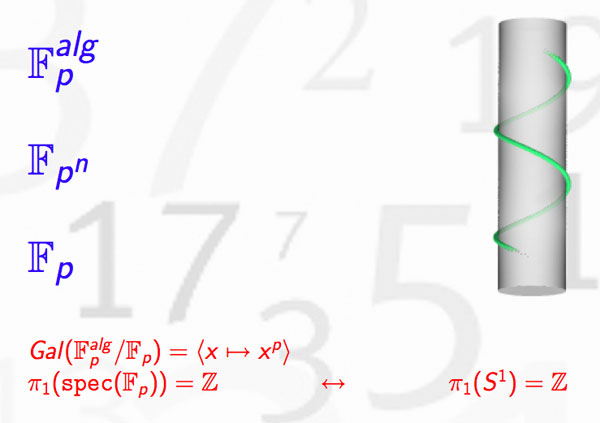In recent months new evidence has come to light allowing us to settle the genesis of this marvelous idea.

1. The former consensusUntil now, the generally accepted view (see for example the ‘Mazur-dictionary-post’ or Morishita’s expository paper) was that the analogy between knots and primes was first pointed out by Barry Mazur in the middle of the 1960’s when preparing for his lectures at the Summer Conference on Algebraic Geometry, at Bowdoin, in 1966. The lecture notes where later published in 1973 in the Annales of the ENS as ‘Notes on etale cohomology of number fields’.

For further use in this series of posts, please note the acknowledgement at the bottom of the first page, reproduced below : “It gives me pleasure to thank J.-P. Serre for his vigorous editing and his suggestions and corrections, which led to this revised version.”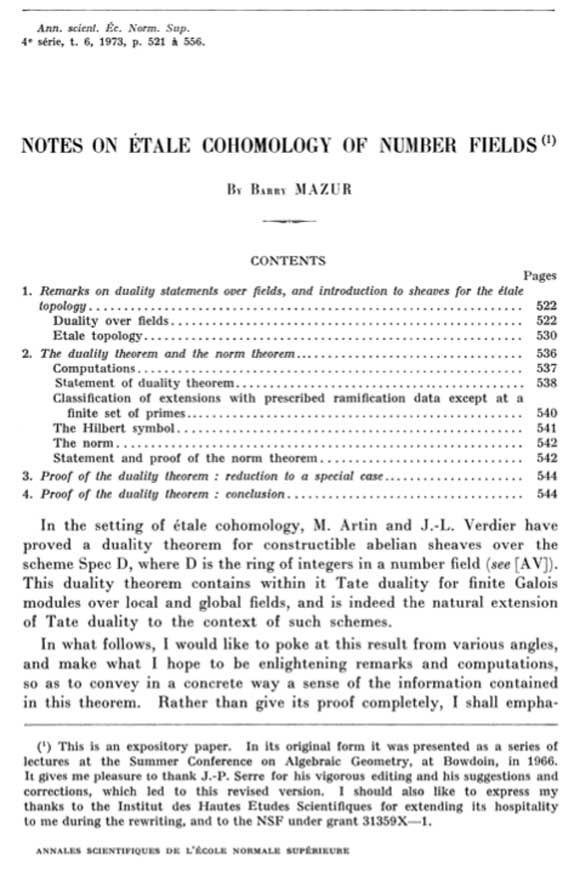Independently, Yuri I. Manin spotted the same analogy at around the same time. However, this point of view was quickly forgotten in favor of the more classical one of viewing number fields as analogous to algebraic function fields of one variable. Subsequently, in the mid 1990’s Mikhail Kapranov and Alexander Reznikov took up the analogy between number fields and 3-manifolds again, and called the resulting study arithmetic topology.

2. The new evidence

On december 13th 2010, David Feldman posted a MathOverflow-question Mazur’s unpublished manuscript on primes and knots?. He wrote : “The story of the analogy between knots and primes, which now has a literature, started with an unpublished note by Barry Mazur. I’m not absolutely sure this is the one I mean, but in his paper, Analogies between group actions on 3-manifolds and number fields, Adam Sikora cites B. Mazur, Remarks on the Alexander polynomial, unpublished notes.

Two months later, on february 15th David Feldman suddenly found the missing preprint in his mail-box and made it available. The preprint is now also available from Barry Mazur’s website. Mazur adds the following comment :

“In 1963 or 1964 I wrote an article Remarks on the Alexander Polynomial [PDF] about the analogy between knots in the three-dimensional sphere and prime numbers (and, correspondingly, the relationship between the Alexander polynomial and Iwasawa Theory). I distributed some copies of my article but never published it, and I misplaced my own copy. In subsequent years I have had many requests for my article and would often try to search through my files to find it, but never did. A few weeks ago Minh-Tri Do asked me for my article, and when I said I had none, he very kindly went on the web and magically found a scanned copy of it. I’m extremely grateful to Minh-Tri Do for his efforts (and many thanks, too, to David Feldman who provided the lead).”The opening paragraph of this unpublished preprint contains a major surprise!

Mazur points to David Mumford as the originator of the ‘primes-are-knots’ idea : “Mumford has suggested a most elegant model as a geometric interpretation of the above situation : $\pmb{spec}(\mathbb{Z}/p\mathbb{Z})$ is like a one-dimensional knot in $\pmb{spec}(\mathbb{Z})$ which is like a simply connected three-manifold.”In a later post we will show that one can even pinpoint the time and place when and where this analogy was first dreamed-up to within a few days and a couple of miles.

For the impatient among you, have a sneak preview of the cradle of birth of the primes=knots idea…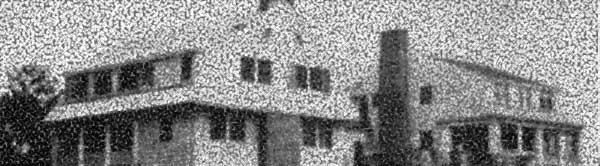In view or recents events & comments, some changes have been made or will be made shortly :

categories : Sanitized the plethora of wordpress-categories to which posts belong. At the moment there are just 5 categories : ‘stories’ and ‘web’ (for all posts with low math-content) and three categories ‘level1’, ‘level2’ and ‘level3’, loosely indicating the math-difficulty of a post.

MathJax : After years of using LatexRender and WP-Latex, we’ll change to MathJax from now on. I’ll try to convert older posts as soon as possible. (Update : did a global search and replace. ‘Most’ LaTeX works, major exceptions being matrices and xymatrix commands. I’ll try to fix those later with LatexRender.)

theme : The next couple of days, the layout of this site may change randomly as I’ll be trying out things with the Swift wordpress theme. Hopefully, this will converge to a new design by next week.

name : Neverendingbooks will be renamed to something more math-related. Clearly, the new name will depend on the topics to be covered. On the main index page a pop-up poll will appear in the lower right-hand corner after 10 seconds. Please fill in the topics you’d like us to cover (no name or email required).

This poll will close on friday 21st at 12 CET and its outcome will influence name/direction of this blog. Use it also if you have a killer newname-suggestion. Among the responses so far, a funnier one : “An intro to, or motivation for non-commutative geometry, aimed at undergraduates. As a rule, I’d take what you think would be just right for undergrads, and then trim it down a little more.”

guest-posts : If you’d like to be a guest-blogger here at irregular times, please contact me. The first guest-post will be on noncommutative topology and the interpretation of quantum physics, and will appear soon. So, stay tuned…

Sunday january 2nd around 18hr NeB-stats went crazy.

Referrals clarified that the post ‘What is the knot associated to a prime?’ was picked up at Reddit/math and remained nr.1 for about a day.

Now, the dust has settled, so let’s learn from the experience.A Reddit-mention is to a blog what doping is to a sporter.

You get an immediate boost in the most competitive of all blog-stats, the number of unique vistors (blue graph), but is doesn’t result in a long-term effect, and, it may even be harmful to more essential blog-stats, such as the average time visitors spend on your site (yellow graph).

For NeB the unique vistors/day fluctuate normally around 300, but peaked to 1295 and 1733 on the ‘Reddit-days’. In contrast, the avg. time on site is normally around 3 minutes, but dropped the same days to 44 and 30 seconds!

Whereas some of the Reddits spend enough time to read the post and comment on it, the vast majority zap from one link to the next. Having monitored the Reddit/math page for two weeks, I’m convinced that post only made it because it was visually pretty good. The average Reddit/math-er is a viewer more than a reader…

So, should I go for shorter, snappier, more visual posts?

Let’s compare Reddits to those coming from the three sites giving NeB most referrals : Google search, MathOverflow and Wikipedia.This is the traffic coming from Reddit/math, as always the blue graph are the unique visitors, the yellow graph their average time on site, blue-scales to the left, yellow-scales to the right.

Here’s the same graph for Google search. The unique visitors/day fluctuate around 50 and their average time on site about 2 minutes.The math-related search terms most used were this month : ‘functor of point approach’, ‘profinite integers’ and ‘bost-connes sytem’.

More rewarding to me are referrals from MathOverflow.The number of visitors depends on whether the MathO-questions made it to the front-page (for example, the 80 visits on december 15, came from the What are dessins d’enfants?-topic getting an extra comment that very day, and having two references to NeB-posts : The best rejected proposal ever and Klein’s dessins d’enfant and the buckyball), but even older MathO-topics give a few referrals a day, and these people sure take their time reading the posts (+ 5 minutes).

Other MathO-topics giving referrals this month were Most intricate and most beautiful structures in mathematics (linking to Looking for F-un), What should be learned in a first serious schemes course? (linking to Mumford’s treasure map (btw. one of the most visited NeB-posts ever)), How much of scheme theory can you visualize? (linking again to Mumford’s treasure map) and Approaches to Riemann hypothesis using methods outside number theory (linking to the Bost-Connes series).

Finally, there’s Wikipediagiving 5 to 10 referrals a day, with a pretty good time-on-site average (around 4 minutes, peaking to 12 minutes). It is rewarding to see NeB-posts referred to in as diverse Wikipedia-topics as ‘Fifteen puzzle’, ‘Field with one element’, ‘Evariste Galois’, ‘ADE classification’, ‘Monster group’, ‘Arithmetic topology’, ‘Dessin d’enfant’, ‘Groupoid’, ‘Belyi’s theorem’, ‘Modular group’, ‘Cubic surface’, ‘Esquisse d’un programme’, ‘N-puzzle’, ‘Shabat polynomial’ and ‘Mathieu group’.

What lesson should be learned from all this data? Should I go for shorter, snappier and more visual posts, or should I focus on the small group of visitors taking their time reading through a longer post, and don’t care about the appallingly high bounce rate the others cause?

Sometimes a MathOverflow question gets deleted before I can post a reply…

Yesterday (New-Year) PD1&2 were visiting, so I merely bookmarked the What is the knot associated to a prime?-topic, promising myself to reply to it this morning, only to find out that the page no longer exists.

From what I recall, the OP interpreted one of my slides of the April 1st-Alumni talk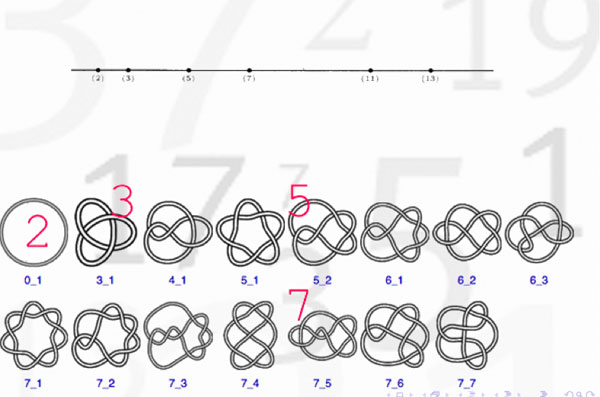as indicating that there might be a procedure to assign to a prime number a specific knot. Here’s the little I know about this :

Artin-Verdier duality in etale cohomology suggests that $Spec(\mathbb{Z})$ is a 3-dimensional manifold, as Barry Mazur pointed out in this paper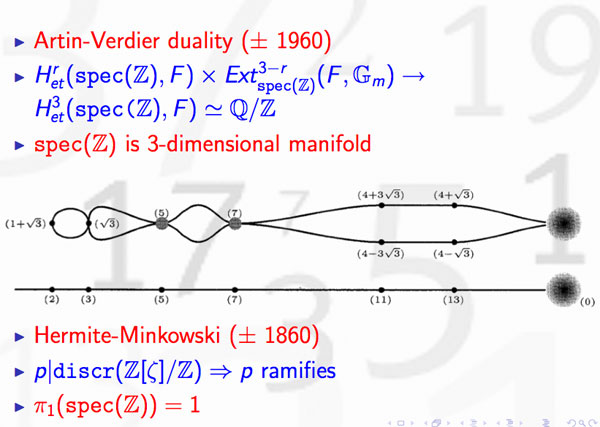The theory of discriminants shows that there are no non-trivial global etale extensions of $Spec(\mathbb{Z})$, whence its (algebraic) fundamental group should be trivial. By Poincare-Perelman this then implies that one should view $Spec(\mathbb{Z})$ as the three-sphere $S^3$. Note that there is no ambiguity in this direction. However, as there are other rings of integers in number fields having trivial fundamental group, the correspondence is not perfect.

Okay, but then primes should correspond to certain submanifolds of $S^3$ and as the algebraic fundamental group of $Spec(\mathbb{F}_p)$ is the profinite completion of $\mathbb{Z}$, the first option that comes to mind are circlesHence, primes might be viewed as circles embedded in $S^3$, that is, as knots! But which knots? Well, as far as I know, nobody has a procedure to assign a knot to a prime number, let alone one having p crossings. What is known, however, is that different primes must correspond to different knots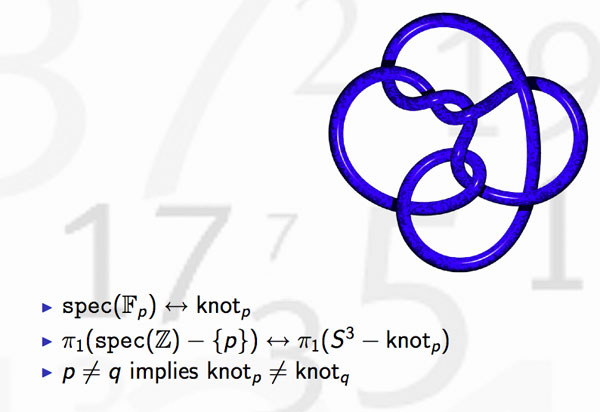because the algebraic fundamental groups of $Spec(\mathbb{Z})- { p }$ differ for distinct primes. This was the statement I wanted to illustrate in the first slide.

But, the story goes a lot further. Knots may be linked and one can detect this by calculating the link-number, which is symmetric in the two knots. In number theory, the Legendre symbol, plays a similar role thanks to quadratic reciprocity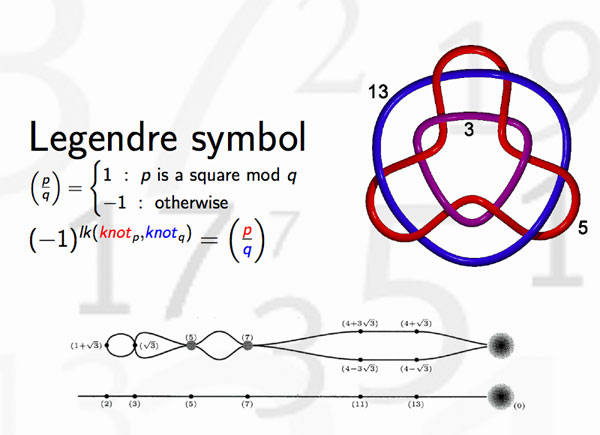and hence we can view the Legendre symbol as indicating whether the knots corresponding to different primes are linked or not. Whereas it is natural in knot theory to investigate whether collections of 3, 4 or 27 knots are intricately linked (or not), few people would consider the problem whether one collection of 27 primes differs from another set of 27 primes worthy of investigation.

There’s one noteworthy exception, the Redei symbol which we can now view as giving information about the link-behavior of the knots associated to three different primes. For example, one can hunt for prime-triples whose knots link as the Borromean rings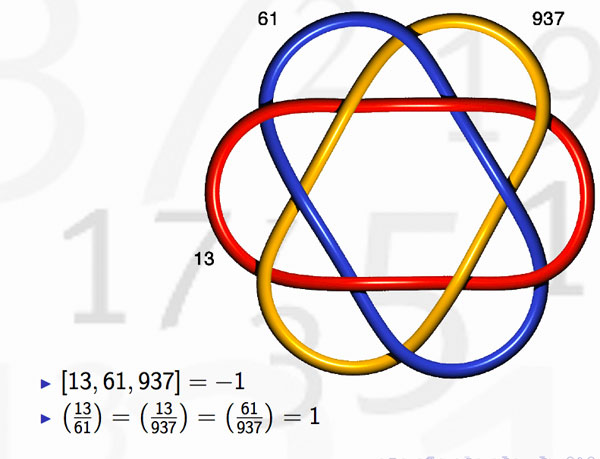(note that the knots corresponding to the three primes are not the unknot but more complicated). Here’s where the story gets interesting : in number-theory one would like to discover ‘higher reciprocity laws’ (for collections of n prime numbers) by imitating higher-link invariants in knot-theory. This should be done by trying to correspond filtrations on the fundamental group of the knot-complement to that of the algebraic fundamental group of $Spec(\mathbb{Z})-{ p }$ This project is called arithmetic topology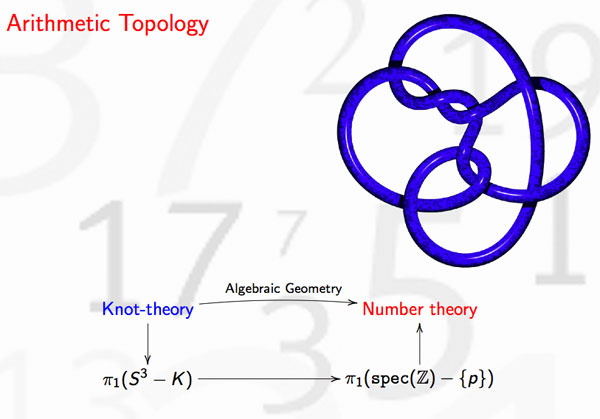Perhaps I should make a pod- or vod-cast of that 20 minute talk, one day…

A few MathOverflow threads I bookmarked in 2010 for various reasons.

This is a belated response to a Math-Overflow exchange between Thomas Riepe and Chandan Singh Dalawat asking for a possible connection between Connes’ noncommutative geometry approach to the Riemann hypothesis and the Langlands program.

Here’s the punchline : a large chunk of the Connes-Marcolli book Noncommutative Geometry, Quantum Fields and Motives can be read as an exploration of the noncommutative boundary to the Langlands program (at least for $GL_1$ and $GL_2$ over the rationals $\mathbb{Q}$).

Recall that Langlands for $GL_1$ over the rationals is the correspondence, given by the Artin reciprocity law, between on the one hand the abelianized absolute Galois group

$Gal(\overline{\mathbb{Q}}/\mathbb{Q})^{ab} = Gal(\mathbb{Q}(\mu_{\infty})/\mathbb{Q}) \simeq \hat{\mathbb{Z}}^*$

and on the other hand the connected components of the idele classes

$\mathbb{A}^{\ast}_{\mathbb{Q}}/\mathbb{Q}^{\ast} = \mathbb{R}^{\ast}_{+} \times \hat{\mathbb{Z}}^{\ast}$

The locally compact Abelian group of idele classes can be viewed as the nice locus of the horrible quotient space of adele classes $\mathbb{A}_{\mathbb{Q}}/\mathbb{Q}^{\ast}$. There is a well-defined map

$\mathbb{A}_{\mathbb{Q}}’/\mathbb{Q}^{\ast} \rightarrow \mathbb{R}_{+} \qquad (x_{\infty},x_2,x_3,\ldots) \mapsto | x_{\infty} | \prod | x_p |_p$

from the subset $\mathbb{A}_{\mathbb{Q}}’$ consisting of adeles of which almost all terms belong to $\mathbb{Z}_p^{\ast}$. The inverse image of this map over $\mathbb{R}_+^{\ast}$ are precisely the idele classes $\mathbb{A}^{\ast}_{\mathbb{Q}}/\mathbb{Q}^{\ast}$. In this way one can view the adele classes as a closure, or ‘compactification’, of the idele classes.

This is somewhat reminiscent of extending the nice action of the modular group on the upper-half plane to its badly behaved action on the boundary as in the Manin-Marcolli cave post.

The topological properties of the fiber over zero, and indeed of the total space of adele classes, are horrible in the sense that the discrete group $\mathbb{Q}^*$ acts ergodically on it, due to the irrationality of $log(p_1)/log(p_2)$ for primes $p_i$. All this is explained well (in the semi-local case, that is using $\mathbb{A}_Q’$ above) in the Connes-Marcolli book (section 2.7).

In much the same spirit as non-free actions of reductive groups on algebraic varieties are best handled using stacks, such ergodic actions are best handled by the tools of noncommutative geometry. That is, one tries to get at the geometry of $\mathbb{A}_{\mathbb{Q}}/\mathbb{Q}^{\ast}$ by studying an associated non-commutative algebra, the skew-ring extension of the group-ring of the adeles by the action of $\mathbb{Q}^*$ on it. This algebra is known to be Morita equivalent to the Bost-Connes algebra which is the algebra featuring in Connes’ approach to the Riemann hypothesis.

It shouldn’t thus come as a major surprise that one is able to recover the other side of the Langlands correspondence, that is the Galois group $Gal(\mathbb{Q}(\mu_{\infty})/\mathbb{Q})$, from the Bost-Connes algebra as the symmetries of certain states.

In a similar vein one can read the Connes-Marcolli $GL_2$-system (section 3.7 of their book) as an exploration of the noncommutative closure of the Langlands-space $GL_2(\mathbb{A}_{\mathbb{Q}})/GL_2(\mathbb{Q})$.

At the moment I’m running a master-seminar noncommutative geometry trying to explain this connection in detail. But, we’re still in the early phases, struggling with the topology of ideles and adeles, reciprocity laws, L-functions and the lot. Still, if someone is interested I might attempt to post some lecture notes here.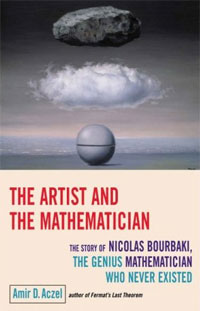Over the week-end I read The artist and the mathematician (subtitle : The story of Nicolas Bourbaki, the genius mathematician who never existed) by Amir D. Aczel.

Whereas the central character of the book should be Bourbaki, it focusses more on two of Bourbaki’s most colorful members, André Weil and Alexander Grothendieck, and the many stories and myths surrounding them.

The opening chapter (‘The Disappearance’) describes the Grothendieck’s early years (based on the excellent paper by Allyn Jackson Comme Appelé du Néant ) and his disappearance in the Pyrenees in the final years of last century. The next chapter (‘An Arrest in Finland’) recount the pre-WW2 years of Weil and the myth of his arrest in Finland and his near escape from execution (based on Weil’s memoires The Apprenticeship of a Mathematician). Chapter seven (‘The Café’) describes the first 10 proto-Bourbaki meetings following closely the study ‘A Parisian Café and Ten Proto-Bourbaki Meetings (1934-1935)‘ by Liliane Beaulieu. Etc. etc.

All the good ‘Bourbaki’-stories get a place in this book, not always historically correct. For example, on page 90 it is suggested that all of the following jokes were pulled at the Besse-conference, July 1935 : the baptizing of Nicolas, the writing of the Comptes-Rendus paper, the invention of the Bourbaki-daughter Betti and the printing of the wedding invitation card. In reality, all of these date from much later, the first two from the autumn of 1935, the final two no sooner than april 1939…

One thing I like about this book is the connection it makes with other disciplines, showing the influence of Bourbaki’s insistence on ‘structuralism’ in fields as different as philosophy, linguistics, anthropology and literary criticism. One example being Weil’s group-theoretic solution to the marriage-rules problem in tribes of Australian aborigines studied by Claude Lévi-Strauss, another the literary group Oulipo copying Bourbaki’s work-method.

Another interesting part is Aczel’s analysis of Bourbaki’s end. In the late 50ties, Grothendieck tried to convince his fellow Bourbakis to redo their work on the foundations of mathematics, changing these from set theory to category theory. He failed as others felt that the foundations had already been laid and there was no going back. Grothendieck left, and Bourbaki would gradually decline following its refusal to accept new methods. In Grothendieck’s own words (in “Promenade” 63, n. 78, as translated by Aczel) :

“Additionally, since the 1950s, the idea of structure has become passé, superseded by the influx of new ‘categorical’ methods in certain of the most dynamical areas of mathematics, such as topology or algebraic geometry. (Thus, the notion of ‘topos’ refuses to enter into the ‘Bourbaki sack’ os structures, decidedly already too full!) In making this decision, in full cognizance, not to engage in this revision, Bourbaki has itself renounced its initial ambition, which has been to furnish both the foundations and the basic language for all of modern mathematics.”

Finally, it is interesting to watch Aczel’s own transformation throughout the book, from slavishly copying the existing Weil-myths and pranks at the beginning of the book, to the following harsh criticism on Weil, towards the end (p. 209) :

“From other information in his autobiography, one gets the distinct impression that Weil was infatuated with the childish pranks of ‘inventing’ a person who never existed, creating for him false papers and a false identity, complete with a daughter, Betti, who even gets married, parents and relatives, and membership in a nonexistent Academy of Sciences of the nonexistent nation of Polvedia (sic).
Weil was so taken with these activities that he even listed, as his only honor by the time of his death ‘Member, Poldevian Academy of Sciences’. It seems that Weil could simply not go beyond these games: he could not grasp the deep significance and power of the organization he helped found. He was too close, and thus unable to see the great achievements Bourbaki was producing and to acknowledge and promote these achievements. Bourbaki changed the way we do mathematics, but Weil really saw only the pranks and the creation of a nonexistent person.”

Judging from my own reluctance to continue with the series on the Bourbaki code, an overdose reading about Weil’s life appears to have this effect on people…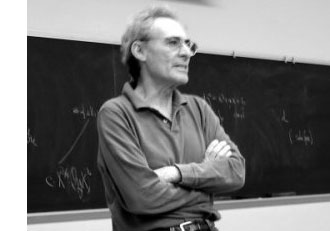In the previous posts, we have depicted the ‘arithmetic line’, that is the prime numbers, as a ‘line’ and individual primes as ‘points’.

However, sometime in the roaring 60-ties, Barry Mazur launched the crazy idea of viewing the affine spectrum of the integers, $\mathbf{spec}(\mathbb{Z})$, as a 3-dimensional manifold and prime numbers themselves as knots in this 3-manifold…

After a long silence, this idea was taken up recently by Mikhail Kapranov and Alexander Reznikov (1960-2003) in a talk at the MPI-Bonn in august 1996. Pieter Moree tells the story in his recollections about Alexander (Sacha) Reznikov in Sipping Tea with Sacha : “Sasha’s paper is closely related to his paper where the analogy of covers of three-manifolds and class field theory plays a big role (an analogy that was apparently first noticed by B. Mazur). Sasha and Mikhail Kapranov (at the time also at the institute) were both very interested in this analogy. Eventually, in August 1996, Kapranov and Reznikov both lectured on this (and I explained in about 10 minutes my contribution to Reznikov’s proof). I was pleased to learn some time ago that this lecture series even made it into the literature, see Morishita’s ‘On certain analogies between knots and primes’ J. reine angew. Math 550 (2002) 141-167.”

Here’s a part of what is now called the Kapranov-Reznikov-Mazur dictionary :What is the rationale behind this dictionary? Well, it all has to do with trying to make sense of the (algebraic) fundamental group $\pi_1^{alg}(X)$ of a general scheme $X$. Recall that for a manifold $M$ there are two different ways to define its fundamental group $\pi_1(M)$ : either as the closed loops in a given basepoint upto homotopy or as the automorphism group of the universal cover $\tilde{M}$ of $M$.

For an arbitrary scheme the first definition doesn’t make sense but we can use the second one as we have a good notion of a (finite) cover : an etale morphism $Y \rightarrow X$ of the scheme $X$. As they form an inverse system, we can take their finite automorphism groups $Aut_X(Y)$ and take their projective limit along the system and call this the algebraic fundamental group $\pi^{alg}_1(X)$.

Hendrik Lenstra has written beautiful course notes on ‘Galois theory for schemes’ on all of this starting from scratch. Besides, there are also two video-lectures available on this at the MSRI-website : Etale fundamental groups 1 by H.W. Lenstra and Etale fundamental groups 2 by F. Pop.

But, what is the connection with the ‘usual’ fundamental group in case both of them can be defined? Well, by construction the algebraic fundamental group is always a profinite group and in the case of manifolds it coincides with the profinite completion of the standard fundamental group, that is,
$\pi^{alg}_1(M) \simeq \widehat{\pi_1(M)}$ (recall that the cofinite completion is the projective limit of all finite group quotients).

Right, so all we have to do to find a topological equivalent of an algebraic scheme is to compute its algebraic fundamental group and find an existing topological space of which the profinite completion of its standard fundamental group coincides with our algebraic fundamental group. An example : a prime number $p$ (as a ‘point’ in $\mathbf{spec}(\mathbb{Z})$) is the closed subscheme $\mathbf{spec}(\mathbb{F}_p)$ corresponding to the finite field $\mathbb{F}_p = \mathbb{Z}/p\mathbb{Z}$. For any affine scheme of a field $K$, the algebraic fundamental group coincides with the absolute Galois group $Gal(\overline{K}/K)$. In the case of $\mathbb{F}_p$ we all know that this abslute Galois group is isomorphic with the profinite integers $\hat{\mathbb{Z}}$. Now, what is the first topological space coming to mind having the integers as its fundamental group? Right, the circle $S^1$. Hence, in arithmetic topology we view prime numbers as topological circles, that is, as knots in some bigger space.

But then, what is this bigger space? That is, what is the topological equivalent of $\mathbf{spec}(\mathbb{Z})$? For this we have to go back to Mazur’s original paper Notes on etale cohomology of number fields in which he gives an Artin-Verdier type duality theorem for the affine spectrum $X=\mathbf{spec}(D)$ of the ring of integers $D$ in a number field. More precisely, there is a non-degenerate pairing $H^r_{et}(X,F) \times Ext^{3-r}_X(F, \mathbb{G}_m) \rightarrow H^3_{et}(X,F) \simeq \mathbb{Q}/\mathbb{Z}$ for any constructible abelian sheaf $F$. This may not tell you much, but it is a ‘sort of’ Poincare-duality result one would have for a compact three dimensional manifold.

Ok, so in particular $\mathbf{spec}(\mathbb{Z})$ should be thought of as a 3-dimensional compact manifold, but which one? For this we have to compute the algebraic fundamental group. Fortunately, this group is trivial as there are no (non-split) etale covers of $\mathbf{spec}(\mathbb{Z})$, so the corresponding 3-manifold should be simple connected… but wenow know that this has to imply that the manifold must be $S^3$, the 3-sphere! Summarizing : in arithmetic topology, prime numbers are knots in the 3-sphere!

More generally (by the same arguments) the affine spectrum $\mathbf{spec}(D)$ of a ring of integers can be thought of as corresponding to a closed oriented 3-dimensional manifold $M$ (which is a cover of $S^3$) and a prime ideal $\mathfrak{p} \triangleleft D$ corresponds to a knot in $M$.

But then, what is an ideal $\mathfrak{a} \triangleleft D$? Well, we have unique factorization of ideals in $D$, that is, $\mathfrak{a} = \mathfrak{p}_1^{n_1} \ldots \mathfrak{p}_k^{n_k}$ and therefore $\mathfrak{a}$ corresponds to a link in $M$ of which the constituent knots are the ones corresponding to the prime ideals $\mathfrak{p}_i$.

And we can go on like this. What should be an element $w \in D$? Well, it will be an embedded surface $S \rightarrow M$, possibly with a boundary, the boundary being the link corresponding to the ideal $\mathfrak{a} = Dw$ and Seifert’s algorithm tells us how we can produce surfaces having any prescribed link as its boundary. But then, in particular, a unit $w \in D^*$ should correspond to a closed surface in $M$.

And all these analogies carry much further : for example the class group of the ring of integers $Cl(D)$ then corresponds to the torsion part $H_1(M,\mathbb{Z})_{tor}$ because principal ideals $Dw$ are trivial in the class group, just as boundaries of surfaces $\partial S$ vanish in $H_1(M,\mathbb{Z})$. Similarly, one may identify the unit group $D^*$ with $H_2(M,\mathbb{Z})$… and so on, and on, and on…

More links to papers on arithmetic topology can be found in John Baez’ week 257 or via here.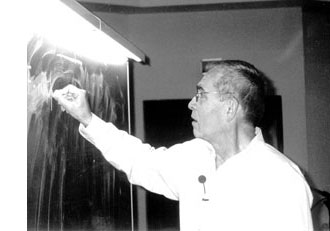Mumford’s drawing has a clear emphasis on the vertical direction. The set of all vertical lines corresponds to taking the fibers of the natural ‘structural morphism’ : $\pi~:~\mathbf{spec}(\mathbb{Z}[t]) \rightarrow \mathbf{spec}(\mathbb{Z})$ coming from the inclusion $\mathbb{Z} \subset \mathbb{Z}[t]$. That is, we consider the intersection $P \cap \mathbb{Z}$ of a prime ideal $P \subset \mathbb{Z}[t]$ with the subring of constants.

Two options arise : either $P \cap \mathbb{Z} \not= 0$, in which case the intersection is a principal prime ideal $~(p)$ for some prime number $p$ (and hence $P$ itself is bigger or equal to $p\mathbb{Z}[t]$ whence its geometric object is contained in the vertical line $\mathbb{V}((p))$, the fiber $\pi^{-1}((p))$ of the structural morphism over $~(p)$), or, the intersection $P \cap \mathbb{Z}[t] = 0$ reduces to the zero ideal (in which case the extended prime ideal $P \mathbb{Q}[x] = (q(x))$ is a principal ideal of the rational polynomial algebra $\mathbb{Q}[x]$, and hence the geometric object corresponding to $P$ is a horizontal curve in Mumford’s drawing, or is the whole arithmetic plane itself if $P=0$).

Because we know already that any ‘point’ in Mumford’s drawing corresponds to a maximal ideal of the form $\mathfrak{m}=(p,f(x))$ (see last time), we see that every point lies on precisely one of the set of all vertical coordinate axes corresponding to the prime numbers ${~\mathbb{V}((p)) = \mathbf{spec}(\mathbb{F}_p[x]) = \pi^{-1}((p))~}$. In particular, two different vertical lines do not intersect (or, in ringtheoretic lingo, the ‘vertical’ prime ideals $p\mathbb{Z}[x]$ and $q\mathbb{Z}[x]$ are comaximal for different prime numbers $p \not= q$).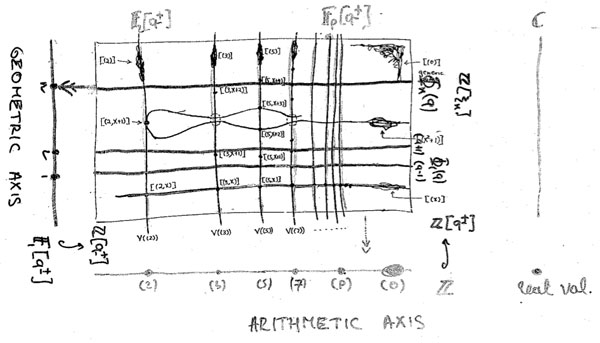That is, the structural morphism is a projection onto the “arithmetic axis” (which is $\mathbf{spec}(\mathbb{Z})$) and we get the above picture. The extra vertical line to the right of the picture is there because in arithmetic geometry it is customary to include also the archimedean valuations and hence to consider the ‘compactification’ of the arithmetic axis $\mathbf{spec}(\mathbb{Z})$ which is $\overline{\mathbf{spec}(\mathbb{Z})} = \mathbf{spec}(\mathbb{Z}) \cup { v_{\mathbb{R}} }$.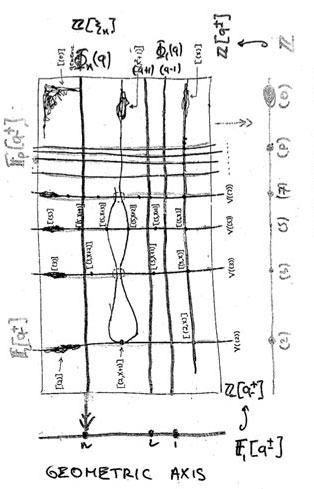Yuri I. Manin is advocating for years the point that we should take the terminology ‘arithmetic surface’ for $\mathbf{spec}(\mathbb{Z}[x])$ a lot more seriously. That is, there ought to be, apart from the projection onto the ‘z-axis’ (that is, the arithmetic axis $\mathbf{spec}(\mathbb{Z})$) also a projection onto the ‘x-axis’ which he calls the ‘geometric axis’.

But then, what are the ‘points’ of this geometric axis and what are their fibers under this second projection?

We have seen above that the vertical coordinate line over the prime number $~(p)$ coincides with $\mathbf{spec}(\mathbb{F}_p[x])$, the affine line over the finite field $\mathbb{F}_p$. But all of these different lines, for varying primes $p$, should project down onto the same geometric axis. Manin’s idea was to take therefore as the geometric axis the affine line $\mathbf{spec}(\mathbb{F}_1[x])$, over the virtual field with one element, which should be thought of as being the limit of the finite fields $\mathbb{F}_p$ when $p$ goes to one!

How many points does $\mathbf{spec}(\mathbb{F}_1[x])$ have? Over a virtual object one can postulate whatever one wants and hope for an a posteriori explanation. $\mathbb{F}_1$-gurus tell us that there should be exactly one point of size n on the affine line over $\mathbb{F}_1$, corresponding to the unique degree n field extension $\mathbb{F}_{1^n}$. However, it is difficult to explain this from the limiting perspective…

Over a genuine finite field $\mathbb{F}_p$, the number of points of thickness $n$ (that is, those for which the residue field is isomorphic to the degree n extension $\mathbb{F}_{p^n}$) is equal to the number of monic irreducible polynomials of degree n over $\mathbb{F}_p$. This number is known to be $\frac{1}{n} \sum_{d | n} \mu(\frac{n}{d}) p^d$ where $\mu(k)$ is the Moebius function. But then, the limiting number should be $\frac{1}{n} \sum_{d | n} \mu(\frac{n}{d}) = \delta_{n1}$, that is, there can only be one point of size one…

Alternatively, one might consider the zeta function counting the number $N_n$ of ideals having a quotient consisting of precisely $p^n$ elements. Then, we have for genuine finite fields $\mathbb{F}_p$ that $\zeta(\mathbb{F}_p[x]) = \sum_{n=0}^{\infty} N_n t^n = 1 + p t + p^2 t^2 + p^3 t^3 + \ldots$, whence in the limit it should become
$1+t+t^2 +t^3 + \ldots$ and there is exactly one ideal in $\mathbb{F}_1[x]$ having a quotient of cardinality n and one argues that this unique quotient should be the unique point with residue field $\mathbb{F}_{1^n}$ (though it might make more sense to view this as the unique n-fold extension of the unique size-one point $\mathbb{F}_1$ corresponding to the quotient $\mathbb{F}_1[x]/(x^n)$…)

A perhaps more convincing reasoning goes as follows. If $\overline{\mathbb{F}_p}$ is an algebraic closure of the finite field $\mathbb{F}_p$, then the points of the affine line over $\overline{\mathbb{F}_p}$ are in one-to-one correspondence with the maximal ideals of $\overline{\mathbb{F}_p}[x]$ which are all of the form $~(x-\lambda)$ for $\lambda \in \overline{\mathbb{F}_p}$. Hence, we get the points of the affine line over the basefield $\mathbb{F}_p$ as the orbits of points over the algebraic closure under the action of the Galois group $Gal(\overline{\mathbb{F}_p}/\mathbb{F}_p)$.

‘Common wisdom’ has it that one should identify the algebraic closure of the field with one element $\overline{\mathbb{F}_{1}}$ with the group of all roots of unity $\mathbb{\mu}_{\infty}$ and the corresponding Galois group $Gal(\overline{\mathbb{F}_{1}}/\mathbb{F}_1)$ as being generated by the power-maps $\lambda \rightarrow \lambda^n$ on the roots of unity. But then there is exactly one orbit of length n given by the n-th roots of unity $\mathbb{\mu}_n$, so there should be exactly one point of thickness n in $\mathbf{spec}(\mathbb{F}_1[x])$ and we should then identity the corresponding residue field as $\mathbb{F}_{1^n} = \mathbb{\mu}_n$.

Whatever convinces you, let us assume that we can identify the non-generic points of $\mathbf{spec}(\mathbb{F}_1[x])$ with the set of positive natural numbers ${ 1,2,3,\ldots }$ with $n$ denoting the unique size n point with residue field $\mathbb{F}_{1^n}$. Then, what are the fibers of the projection onto the geometric axis $\phi~:~\mathbf{spec}(\mathbb{Z}[x]) \rightarrow \mathbf{spec}(\mathbb{F}_1[x]) = { 1,2,3,\ldots }$?

These fibers should correspond to ‘horizontal’ principal prime ideals of $\mathbb{Z}[x]$. Manin proposes to consider $\phi^{-1}(n) = \mathbb{V}((\Phi_n(x)))$ where $\Phi_n(x)$ is the n-th cyclotomic polynomial. The nice thing about this proposal is that all closed points of $\mathbf{spec}(\mathbb{Z}[x])$ lie on one of these fibers!

Indeed, the residue field at such a point (corresponding to a maximal ideal $\mathfrak{m}=(p,f(x))$) is the finite field $\mathbb{F}_{p^n}$ and as all its elements are either zero or an $p^n-1$-th root of unity, it does lie on the curve determined by $\Phi_{p^n-1}(x)$.

As a consequence, the localization $\mathbb{Z}[x]_{cycl}$ of the integral polynomial ring $\mathbb{Z}[x]$ at the multiplicative system generated by all cyclotomic polynomials is a principal ideal domain (as all height two primes evaporate in the localization), and, the fiber over the generic point of $\mathbf{spec}(\mathbb{F}_1[x])$ is $\mathbf{spec}(\mathbb{Z}[x]_{cycl})$, which should be compared to the fact that the fiber of the generic point in the projection onto the arithmetic axis is $\mathbf{spec}(\mathbb{Q}[x])$ and $\mathbb{Q}[x]$ is the localization of $\mathbb{Z}[x]$ at the multiplicative system generated by all prime numbers).

Hence, both the vertical coordinate lines and the horizontal ‘lines’ contain all closed points of the arithmetic plane. Further, any such closed point $\mathfrak{m}=(p,f(x))$ lies on the intersection of a vertical line $\mathbb{V}((p))$ and a horizontal one $\mathbb{V}((\Phi_{p^n-1}(x)))$ (if $deg(f(x))=n$).
That is, these horizontal and vertical lines form a coordinate system, at least for the closed points of $\mathbf{spec}(\mathbb{Z}[x])$.

Still, there is a noticeable difference between the two sets of coordinate lines. The vertical lines do not intersect meaning that $p\mathbb{Z}[x]+q\mathbb{Z}[x]=\mathbb{Z}[x]$ for different prime numbers p and q. However, in general the principal prime ideals corresponding to the horizontal lines $~(\Phi_n(x))$ and $~(\Phi_m(x))$ are not comaximal when $n \not= m$, that is, these ‘lines’ may have points in common! This will lead to an exotic new topology on the roots of unity… (to be continued).